# Online Class: Statistics 101\$95.00
no certificate
- OR -
\$120.00
with CEU Certificate*
Start Right Now!
Taking multiple courses? Save with our platinum program.

• 15
Lessons
• 26
Exams &
Assignments
• 3,272
Students
have taken this course
• 35
Hours
average time
• 3.5
CEUs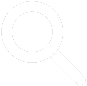## Course Description

Statistics are used in a variety of contexts ranging from scientific experiments to political advertisements and beyond. Because statistics can be used to mislead, an understanding of the topic can be helpful for more than just the mathematical skills that it imparts: knowledge of statistics theory can also be a strong defense against attempts by the unscrupulous to mislead others.

This statistics course introduces the basic concepts of statistical analysis, with a focus on both univariate (single-variable) and bivariate (two-variable) data. The course starts with an introduction to statistics terms and then moves on to organization and display of data. Analysis of univariate data by way of measures of central tendency (such as the mean or average), dispersion (such as the variance), and asymmetry ("skewness") is presented next, followed by an introduction to probability theory.

The relationship of probability to statistics is also discussed, providing students with the tools they need to understand how "chance" plays a role in statistical analysis. Statistical distributions, with a focus on the normal distribution and its uses, are also considered, along with a discussion of bivariate data and linear (least-squares) regression. Finally, the course culminates with a low-level introduction to hypothesis testing. Although this last topic could be a course of its own, the student is provided with enough theory and sufficient practice to conduct analyses of simple statistical hypotheses.

The course assumes a minimal understanding of and proficiency in algebra, but many of the concepts can be understood, and even many of the calculations performed, without an extensive mathematical background. This course is designed for anyone who wants a little more than just a cursory overview of statistics, but who doesn't want to get bogged down in the mathematical theory that underlies it.

Statistics is a subject that has earned a certain amount of notoriety because of its misuse in various contexts. Nevertheless, statistics is a tool that, if used properly, can be of tremendous help in math, science, engineering, history, politics, and numerous other fields. As you study this subject, always keep in mind that statistics is more than just math: it is not simply manipulation of numbers through addition, subtraction, multiplication, division, and other mathematical operations. Statistics also involves language and units: when a statistician (or layman) provides a statistic, it involves a number and a label of some sort. For instance, the number 5.3 is not in and of itself a statistical value; "an average age of 5.3 years," however, is a statistical value. This linguistic aspect of statistics sometimes allows a certain amount of ambiguity that can be misleading. By studying statistics, you will equip yourself to identify and understand both uses and abuses of this tool.

What is Statistics?

Statistics is used for quantifying sets of data such as attributes of a group of people and measurements taken in a laboratory. Consider, for instance, the population of a particular country. The people who reside in that country have varying heights: some are short, some are tall, some are in between. If we wanted to compare the height of this population with that of some other population in a convenient manner, we would not want to compare individual people. Such a task would be burdensome (the number of people in a country might be in the millions or billions) and would not necessarily be particularly helpful as a means of comparing populations as a whole. Instead, we can use an average or median height as the basis for our comparison. These statistical values are single numbers that quantify the data (the heights of a country's population) and that provide a convenient way to express and compare certain characteristics of those data. Part of the goal of this course is to teach you how to select and use statistical tools like averages and medians, as well as a host of others, in assessing and comparing data.

Simply defined, statistics (sometimes colloquially termed "stats") is the study of collecting, analyzing, interpreting, and representing of sets of numerical data. Thus, virtually any field of study that uses numbers can, at least occasionally, involve statistics. Statistics, because it makes extensive use of numbers, is math-intensive, and a decent grasp of basic arithmetic and algebra is required to study this field.

• Completely Online
• Self-Paced
• 6 Months to Complete
• Start Anytime
• PC & Mac Compatible
• Android & iOS Friendly
• Accredited CEUs## Course Lessons

Average Lesson Rating: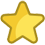#### Lesson 1 - Statistics Terms and Motivation

This lesson will explain why the study of statistics is important, and it will introduce you to some of the key terms and ideas that will be critical to understanding subsequent lessons. 11 Total Points
•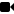Lesson 1 Video A
•Lesson 1 Video B : How to Take an Exam
•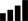Take Survey: Reasons for Taking this Course
•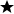Complete Assignment: Introduction
•Complete Exam: Lesson 1 Statistics Terms and Motivation

#### Lesson 2 - Displaying Statistical Data

This lesson will teach you to recognize and construct tabular and graphical representations of data, which is a crucial skill that you will use in later chapters. 9 Total Points
•Lesson 2 Video
•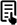Review Article: Concept of Graphical representation of Data
•Complete Exam: Lesson 2 Displaying Statistical Data

#### Lesson 3 - Measures of Central Tendency

In this lesson, you will learn how to describe the "central tendency" of a data set--that is, a number that characterizes the "center" of the data. 13 Total Points
•Lesson 3 Video
•Review 2 Articles: Measures of Central Tendency; Mean and Medain
•Complete: Lesson 3 Exercises
•Complete Exam: Lesson 3 Measures of Central Tendency

#### Lesson 4 - Selecting an Appropriate Measure of Central Tendency

Now that we have studied several different measures of central tendency, we now consider some of the factors that you must take into account when selecting an appropriate measure of central tendency 11 Total Points
•Lesson 4 Video
•Complete Exam: Lesson 4 Selecting an Appropriate Measure of Central Tendency

#### Lesson 5 - Measures of Dispersion

This lesson introduces you to measures of dispersion, which quantify how widely data is spread around the mean. 14 Total Points
•Lesson 5 Video
•Review Article: Standard Deviation and Variance
•Complete: Lesson 5 Exercises
•Complete Exam: Lesson 5 Measures of Dispersion

#### Lesson 6 - Measures of Asymmetry

In this lesson, we will study a measure of asymmetry--skewness--which quantifies how data is "skewed" to either side of the mean. 9 Total Points
•Lesson 6 Video
•Review Article: What is a moment
•Complete Exam: Lesson 6 Measures of Asymmetry

#### Lesson 7 - Other Statistical Measures

This lesson introduces several more miscellaneous statistical measures that can be useful in certain contexts. 14 Total Points
•Lesson 7 Video
•Complete: Lesson 7 Exercises
•Complete Exam: Lesson 7 Other Statistical Measures

#### Lesson 8 - Introduction to Probability I

Although probability theory could constitute a course of its own, a brief presentation of some of the elements of this area is helpful for our study of statistics. 17 Total Points
•Lesson 8 Video
•Complete: Lesson 8 Exercises
•Complete Exam: Lesson 8 Introduction to Probability I

#### Lesson 9 - Introduction to Probability II

This lesson discusses how we can solve probability problems by counting the number outcomes of a particular random experiment. 14 Total Points
•Lesson 9 Video
•Complete: Lesson 9 Exercises
•Complete Exam: Lesson 9 Introduction to Probability II

#### Lesson 10 - Statistical Distributions

This lesson combines what we've learned in both statistics and probability and applies this knowledge to statistical (probability) distributions. 14 Total Points
•Lesson 10 Video
•Complete: Lesson 10 Exercises
•Complete Exam: Lesson 10 Statistical Distributions

#### Lesson 11 - The Normal Distribution

This lesson presents the normal distribution, which is a widely used statistical distribution, as well as how to use it to calculate probabilities. 16 Total Points
•Lesson 11 Video
•Complete: Lesson 11 Exercises
•Complete Exam: Lesson 11 The Normal Distribution

#### Lesson 12 - Bivariate Data

This lesson introduces bivariate data distributions and discusses some related concepts such as scatterplots, marginal distributions, and independence of random variables. 9 Total Points
•Lesson 12 Video
•Complete Exam: Lesson 12 Bivariate Data

#### Lesson 13 - Regression Analysis

This lesson introduces the concept of regression with a focus exclusively on least-squares linear regression. 10 Total Points
•Lesson 13 Video
•Complete Exam: Lesson 13 Regression Analysis

#### Lesson 14 - Introduction to Hypothesis Testing I

This lesson brings together much of what we have learned so far and applies it to statistical hypothesis testing. 13 Total Points
•Lesson 14 Video
•Complete: Lesson 14 Exercises
•Complete Exam: Lesson 14 Introduction to Hypothesis Testing I

#### Lesson 15 - Introduction to Hypothesis Testing II

The final lesson in this course provides a brief overview of the types of errors associated with hypothesis testing and also provides practice problems to reinforce the concepts and skills studied throughout this course. 158 Total Points
•Lesson 15 Video
•Take Poll: How would you rate this course?
•Take Survey: Program Evaluation Follow-up Survey (End of Course)
•Complete: Lesson 15 Exercises
•Complete Exam: Lesson 15 Introduction to Hypothesis Testing II
•Complete: The Final Exam
332
Total Course Points

## Learning Outcomes

By successfully completing this course, students will be able to:
• Define statistics terms.
• Display statistical data.
• Define measures of central tendency.
• Select an appropriate measure of central tendency.
• Define measures of dispersion.
• Describe measures of asymmetry.
• Describe Probability I problems
• Describe and solve Probability II problems.
• Summarize statistical distributions.
• Describe the normal distribution.
• Describe bivariate data.
• Perform regression analysis.
• Describe Hypothesis Testing I and II, and
• Demonstrate mastery of lesson content at levels of 70% or higher.• Document Your Lifelong Learning Achievements
• Earn an Official Certificate Documenting Course Hours and CEUs
• Verify Your Certificate with a Unique Serial Number OnlineCourse Title: Statistics 101
Course Number: 9770453
Lessons Rating: 4.7 / 5 Stars (879 votes)
Languages: English - United States, Canada and other English speaking countries
Category:
Course Type: General Education (Self-Paced, Online Class)
CEU Value: 3.5 IACET CEUs (Continuing Education Units)
CE Accreditation: Universal Class, Inc. has been accredited as an Authorized Provider by the International Association for Continuing Education and Training (IACET).
Assessment Method: Lesson assignments and review exams
Course Author: Dr. Jeffrey Clark, Ph.D.
Instructor: April Cordry-Moore
Syllabus: View Syllabus
Duration: Continuous: Enroll anytime!
Course Fee: \$95.00 (no CEU Certification) || with Online CEU Certification: \$120.00

Course Only
One Course
No Certificate / No CEUs
\$95
for 6 months

Billed once
This course only
 Includes certificate X Includes CEUs X Self-paced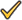Instructor supportTime to complete 6 months No. of courses 1 course
Certificate Course
One Course
Certificate & CEUs
\$120
for 6 months

Billed once
This course only
 Includes certificateIncludes CEUsSelf-pacedInstructor supportTime to complete 6 months No. of courses 1 course
Platinum Yearly
ALL COURSES
Certificates & CEUs
\$189
per year
You save 50%!
Billed once
Includes all 500+ courses
 Includes certificateIncludes CEUsSelf-pacedInstructor supportTime to complete 12 Months No. of courses 500+
Platinum 2 Years
ALL COURSES
Certificates & CEUs
\$299
for 2 years
You save \$427.00!
Billed once
Includes all 500+ courses
 Includes certificateIncludes CEUsSelf-pacedInstructor supportTime to complete 24 Months No. of courses 500+

## Student Testimonials

• "I found that the course resources helpful. I needed it especially for the lesson on normal distriuubtion. I needed to refer to it for the z-scores....Great instructor. Very helpful and responsive." -- Tiffany L.
• "The instructor is an excellent instructor. He simplified a lot of complicated formulas which involve calculus. He also has good examples that make the concepts easier to understand. In addition, he gives challenging assignments and exams. He fully explains all the questions I have missed during exams and assignments." -- Cristeta K.
• "Exellent instructor." -- Angel E.
• "Regression Analysis was most helpful to me....Thanks instructor!" -- Mike A.
• "I knew nothing about Statistics and now I know that I don't want to know more. I enjoyed the class and thought the instructor was very good." -- Donna N.
• "The instructor is wonderful. I would have him as an instructor again." -- Cynthia M.
• "The Instructor was very pleasant, offering words of encouragement when I did poorly on the second exam. He was fairly prompt with grading assignments and answering emails. I would recommend him for this class in the future." -- Jessica S.
• View More Testimonials...